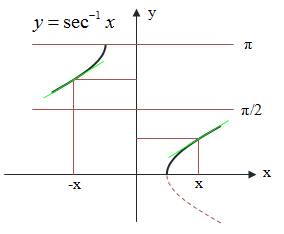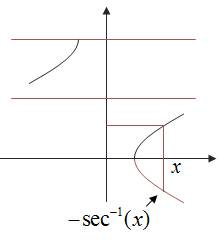# Derivative of an inverse function

• Karol

## Homework Statement

$$\int\frac{dx}{x\sqrt{x^2-1}}=\left\{ \begin{array} {lc} \sec^{-1}(x)+C_1 & {\rm if}~x>1 \\ \sec^{-1}(-x)+C_2 & {\rm if}~x<-1 \end{array} \right.$$
Why the second condition ##\sec^{-1}(-x)+C_2~~{\rm if}~x<-1## ?## Homework Equations

Derivative of inverse secant:
$$(\sec^{-1}(x))'=\frac{1}{x(\pm\sqrt{x^2-1})}$$

## The Attempt at a Solution

The derivative is positive from -∞ to +∞ (the green lines). as i understand i have to make the function ##\sec^{-1}(-x)## positive when x<-1, but if ##y=\sec^{-1}(x)## is defined like in the drawing it is positive alsways

Continuity: ##\sec^{-1}(-x)=\pi-\sec^{-1}(x)## so:
$$\int\frac{dx}{x\sqrt{x^2-1}}=\left\{ \begin{array} {lc} \sec^{-1}(x)+C_1 & {\rm if}~x>1 \\ -\sec^{-1}(x)+C_2 & {\rm if}~x<-1 \end{array} \right.$$
But that gives a negative derivative, if i substitute x<-1 in:
$$\frac{dx}{x\sqrt{x^2-1}}$$If ##x<-1##, then
$$\frac{dx}{x\sqrt{x^2-1}}= \frac{d(-x)}{(-x)\sqrt{(-x)^2-1}} = \frac{d(-x)}{\lvert (-x)\rvert \sqrt{(-x)^2-1}} = d[\sec(-x)]$$

I don't understand the transition:
$$\frac{d(-x)}{(-x)\sqrt{(-x)^2-1}} = \frac{d(-x)}{\lvert (-x)\rvert \sqrt{(-x)^2-1}}$$
Why did (-x) in the denominator became |(-x)|? why is it allowed?

##x## is negative, no?